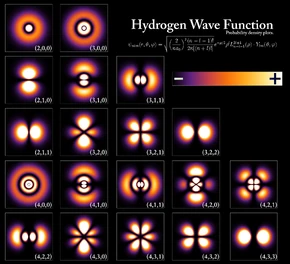303 Pages

## Problem

Consider the one-dimensional, time-dependent probability densityand the current densitywhereand.

Show by calculation that.

Hint: Use the Schrödinger equation and its complex conjugate.## Solution

Calculate the partial derivative of the probability density with respect to.The Schrödinger equation and its complex conjugate can be written asThusCalculate the partial derivative of the current density with respect to.It is clear from the above calculations that, therefore;whereandare the wavefunction and its complex conjugate,is the probability density, andis the current density.